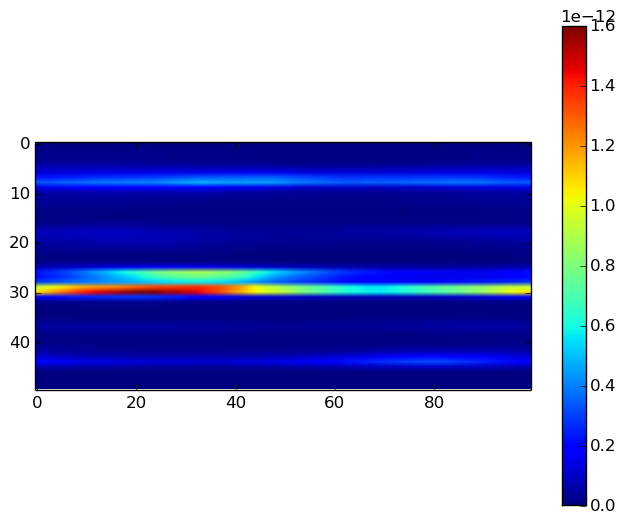# How to generate a log-random permeability field

I was looking for a simple method to generate a log-random permeability field with specified Dykstra-Parsons coefficient and correlation length. I found this webpage with some well-written Matlab codes for the generation of rough surfaces. I'm literally illiterate when it comes to signal processing. All I know is that the Dykstra-Parsons coefficient is defined as $$V_{DP}=\frac{\text{Standard deviation of }\log(k)}{\text{Average of }\log(k)}=\frac{\log(k)_{P50}-\log(k)_{P84.1}}{\log(k)_{P50}}$$ We can rearrange the above equation to obtain the standard deviation of the permeability field: $$\sigma = -\log(1-V_{DP})$$ I used this standard deviation and the average permeability value, $k_{ave}$, to generate a random distribution, and pass it through the filter that I found in the mentioned Matlab codes to generate a log-random permeability field. I showed the result to a Geologist colleague of mine, and he confirmed that it can indeed be considered as an acceptable permeability field. Here's one sample code for a 2D field generation, in Julia. You can easily convert it to Matlab.

In :
function permfieldlogrndg(Nx,Ny,k_avrg,V_dp,clx,cly)
# 2D random field generator:
# hdf: Gaussian
# acf: Gaussian
# The surface has a Gaussian height distribution function
# and Gaussian autocovariance functions
Lx=1.0
X = linspace(-Lx/2,Lx/2,Nx)
Y = linspace(-Lx/2,Lx/2,Ny)'

s = -log(1-V_dp)
mu = log(k_avrg)-s^2/2
Z = s*randn(Nx,Ny)
# Gaussian filter
F = exp(-(X.^2/(clx^2/2.0).+Y.^2/(cly^2/2.0)))
# correlated surface generation including convolution (faltning) and inverse
# Fourier transform and normalizing prefactors
f = 2.0/sqrt(pi)*Lx/sqrt(Nx*Ny)/sqrt(clx)/sqrt(cly)*ifft(fft(Z).*fft(F))
perm = exp(mu+real(f))
end;


Now we can test the code to generate a field on a Nx x Ny grid, an average permeability of k_avrg, a Dykstra-Parsons coefficient V_dp, and correlation length of clx in x direction and cly in y direction.

In :
using PyPlot;

In :
Nx=100
Ny=50
clx=1.0
cly=0.05
k_avg= 1e-12 # [m^2]
V_dp=0.9
k= permfieldlogrndg(Nx,Ny,k_avg,V_dp,clx,cly)
imshow(k')
colorbar()
#tight_layout();The above figure shows a permeability field, for a layered reservoir (correlation length of 1.0 in the x direction). Play a bit with the code, and see what happens. I'll really appreciate your comments about the flaws in this simple code.